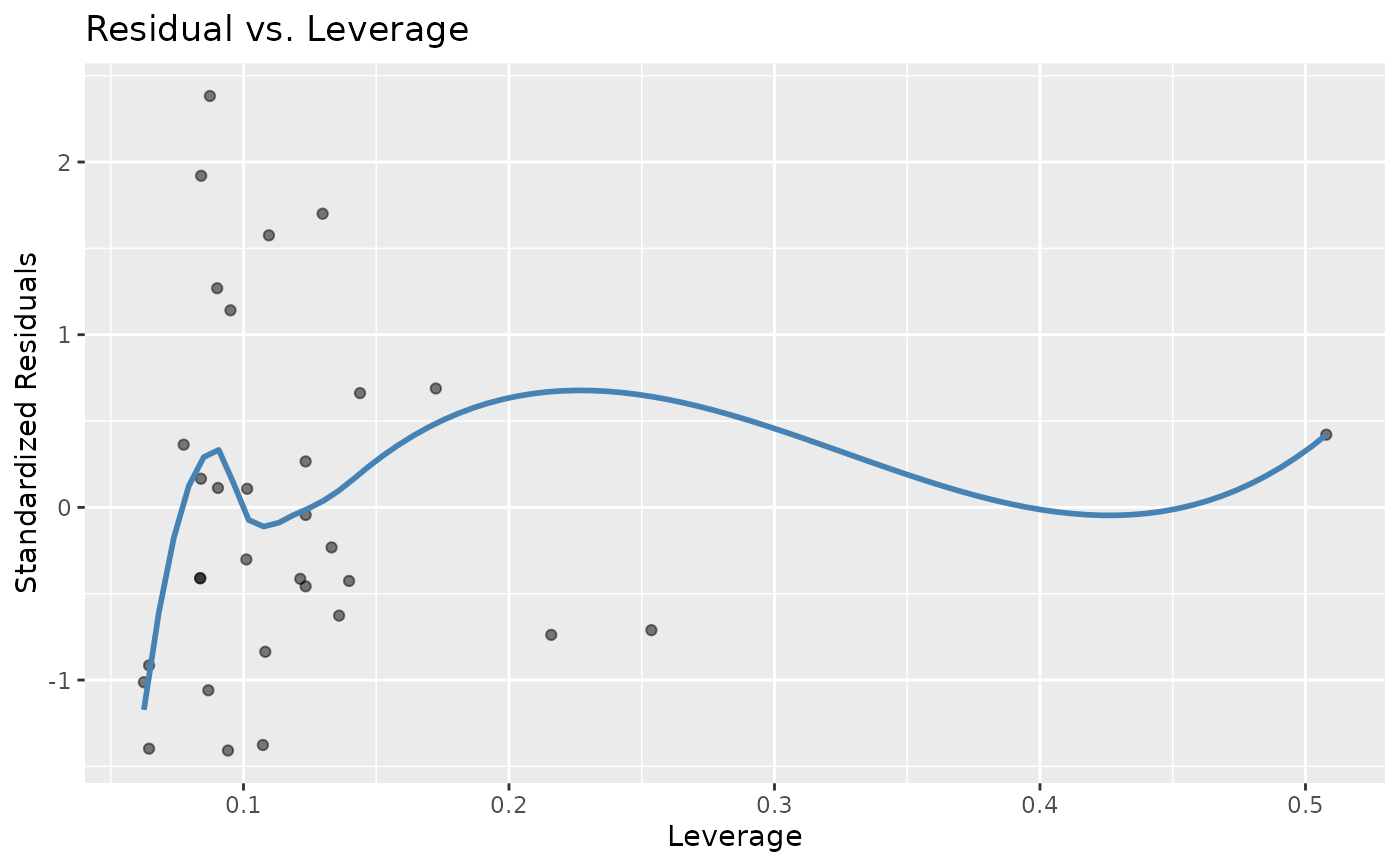Residual vs. leverage plot.

stat_resid_leverage(
alpha = 0.5,
method = "loess",
se = FALSE,
color = "steelblue",
...
)

## Arguments

alpha

method

Method for fitting the line to the points.

se

Keep standard error bands around line?

color

Color of the line.

...

Currently ignored. For extendability.

## Value

A ggplot2 layer for plotting a fitted vs. residual scatter plot.

## Examples

data(mtcars)
model <- lm(mpg ~ cyl + disp + hp, data = mtcars)
ggplot2::ggplot(data = model) + stat_resid_leverage()